Home   »   Important Reasoning Questions For NVS Exam...

# Important Reasoning Questions For NVS Exam | 6th August 2019

The reasoning is the most scoring section in any competitive exam. It also develops or nourishes our critical thinking skills as compared to other subjects. In DSSSBKVSNVS Exam, as a student to score well in this part, you have to start your preparation now because there is negative marking as well. The more you have prepared, the better you score.

Q1. From the given alternatives, select the word which CANNOT be formed using the letters of the given word.
Disbursement
(a) Burst
(b) Sent
(c) Barn
(d) Reid

Q2. In a certain code language, “TURTLE” is written as “RRNRIA”. How is “LOYALS” written in that code language?
(a) ULJOPY
(b) JLUYIO
(c) YOKLJV
(d) VJLOIP

Q3. In a certain code language, ‘x’ represents ‘+’, ‘÷’ represents ‘x’, ‘-‘ represents ‘÷’ and ‘+’ represents ‘-‘. Find out the answer to the following question.
8×10÷20-5+14=
(a) 34
(b) 27
(c) 45
(d) 9

Q4. The following equation is incorrect. Which two signs should be interchanged to correct the equation?
14 + 18 x 40 – 10 ÷ 20 = 66
(a) + and x
(b) + and ÷
(c) – and +
(d) ÷ and –
Q5. If 7 6 = 84, 8Ω7 = 112 and 8Ω4 = 64, then ﬁnd the value of 3Ω4 = ?
(a) 24
(b) 12
(c) 4
(d) 20

Q6. In the following question, select the related number pair from the given alternatives.
16 : 48 : : ? : ?
(a) 25 : 75
(b) 11 : 32
(c) 25 : 64
(d) 49 : 72

Q7. In the following question, select the related letter/letters from the given alternatives.
APR : BQS : : ETV : ?
(a) FVX
(b) FUW
(c) GUX
(d) GVW
Q8. In the following question, select the odd word pair from the given alternatives.
(a) Pen –  Ink
(b) Pen – Writer
(c) Book – Pages
(d) Word –  Letter
Q9. In the following question, four number pairs are given. The number on left side of (–) is related to the number on the right side of (–) with some Logic/Rule/Relation. Three are similar on basis of same Logic/Rule/Relation. Select the odd one out from the given alternatives.
(a) 7 – 14
(b) 11 – 22
(c) 5 – 10
(d) 3 – 8
Q10. In the following question, select the odd letter/letters from the given alternatives.
(a) KRY
(b) DKR
(c) FMT
(d) LVZ
Solutions:
S1. Ans.(c)
Sol. Barn, a is absent in the given word “Disbursement”
S3. Ans.(a)
Sol.
8 × 10 ÷ 20 – 5 + 14 =
8 + 10 ×20 ÷ 5 – 14 = 8 + 10 × 4 – 14 = 34
S4. Ans.(d)
Sol.
14 + 18 × 40 – 10 ÷ 20 = 66
Interchanging ÷ & –
14 + 18 × 40 ÷ 10 – 20
= 14 + 18 × 4 – 20 = 86 -20 = 66
S5. Ans.(a)
Sol.
7 Ω 6 = (7 × 6)2 = 84
8 Ω 7 = (8 × 7)×2 = 112
8 Ω 4 = (8 × 4)×2 = 64
∴ 3 Ω 4 = (3 × 4)2 = 24
S6. Ans.(a)
Sol.
16 × 3 = 48
25 × 3 = 75
S7. Ans.(b)
Sol.
+1 series
S8. Ans.(d)
Sol.
Except for option (b) in all other options 2nd is a part of the 1st.
S9. Ans.(d)
Sol.
Except for option (d) in all other options 2nd one is 2 times the 1st one.
S10. Ans.(d)
Sol.
Except for option (d), rest all are +7 series.

Sharing is caring!

Thank You, Your details have been submitted we will get back to you.
•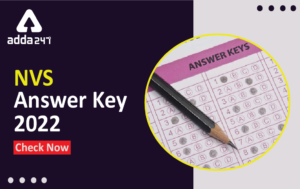NVS Answer Key 2022 For Non Teaching Pos...
•NVS 2022 Salary Structure, Pay Scale For...
•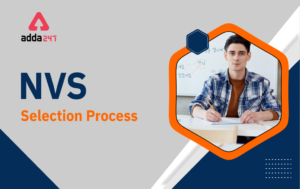NVS Selection Process 2022 & Cut Off...
•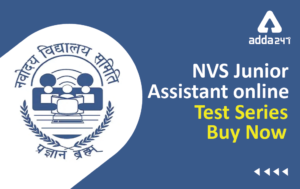Best NVS Online Test Series For Junior S...
•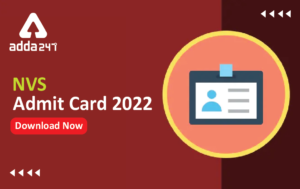NVS Admit Card 2022 Out, Direct Download...
•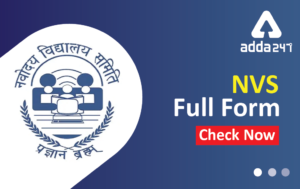NVS Full Form: Full Meaning of NVS in Hi...